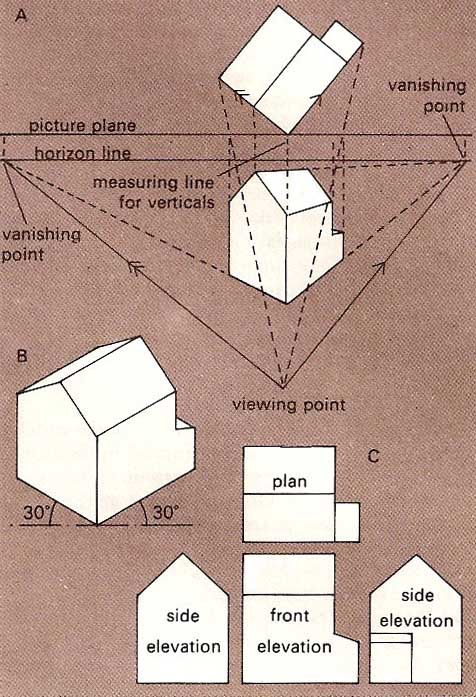A# descriptive geometryThe same object viewed in (A) two-point perspective, (B) isometric projection, and (C) third-angle orthographic projection.

Descriptive geometry is the branch of geometry concerned with the representation of three-dimensional objects on a plane, the basis of architectural and technical drawing. There are two main systems of projection: the perspective view in which the object is projected from point onto a plane (see projective geometry; and orthographic projection, where the object is projected from one plane onto another.

In orthographic projection, two planes are considered, at right angles to each other, the vertical plane and the horizontal plane, intersecting in a line termed the ground line. From each point of the object, perpendiculars are dropped to each of the two planes, giving the horizontal and vertical coordinates of that point. The representation of this on a plane surface is achieved by considering the two planes to be hinged along the ground line so that the horizontal plane may be rotated through 90° to coincide with the vertical, thus providing a graphical representation of the object.